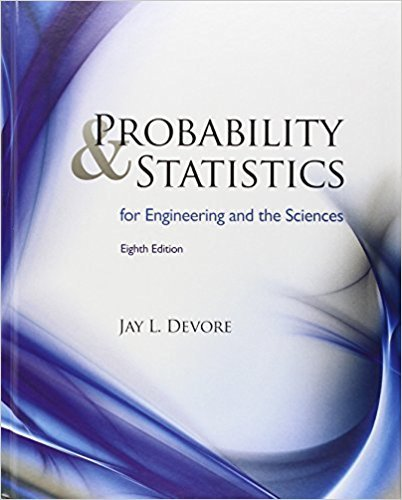# The article from which the data in Exercise 1 was extracted alsogave the accompanying

Chapter 6, Problem 4

(choose chapter or problem)

Get Unlimited Answers! Check out our subscriptions
QUESTION:

The article from which the data in Exercise 1 was extracted alsogave the accompanying strength observations for cylinders:6.1 5.8 7.8 7.1 7.2 9.2 6.6 8.3 7.0 8.37.8 8.1 7.4 8.5 8.9 9.8 9.7 14.1 12.6 11.2Prior to obtaining data, denote the beam strengths by X1, . . . ,Xm and the cylinder strengths by Y1, . . . , Yn. Suppose thatthe Xis constitute a random sample from a distribution withmean 1 and standard deviation s1 and that the Yis form arandom sample (independent of the Xis) from anotherdistribution with mean m2 and standard deviation s2 a. Use rules of expected value to show that is an unbiasedestimator of 1 2. Calculate the estimate for thegiven data.b. Use rules of variance from Chapter 5 to obtain an expressionfor the variance and standard deviation (standarderror) of the estimator in part (a), and then compute theestimated standard error.c. Calculate a point estimate of the ratio s1/s2 of the twostandard deviations.d. Suppose a single beam and a single cylinder are randomlyselected. Calculate a point estimate of the variance of the differenceX Y between beam strength and cylinder strength.

QUESTION:

The article from which the data in Exercise 1 was extracted alsogave the accompanying strength observations for cylinders:6.1 5.8 7.8 7.1 7.2 9.2 6.6 8.3 7.0 8.37.8 8.1 7.4 8.5 8.9 9.8 9.7 14.1 12.6 11.2Prior to obtaining data, denote the beam strengths by X1, . . . ,Xm and the cylinder strengths by Y1, . . . , Yn. Suppose thatthe Xis constitute a random sample from a distribution withmean 1 and standard deviation s1 and that the Yis form arandom sample (independent of the Xis) from anotherdistribution with mean m2 and standard deviation s2 a. Use rules of expected value to show that is an unbiasedestimator of 1 2. Calculate the estimate for thegiven data.b. Use rules of variance from Chapter 5 to obtain an expressionfor the variance and standard deviation (standarderror) of the estimator in part (a), and then compute theestimated standard error.c. Calculate a point estimate of the ratio s1/s2 of the twostandard deviations.d. Suppose a single beam and a single cylinder are randomlyselected. Calculate a point estimate of the variance of the differenceX Y between beam strength and cylinder strength.

a. The estimator for the difference in means is given by = 1 2. By the rules of expected value, E[] = 1 2. As this is an unbiased estimator, we can use this to calculate the estimate for the given data using the sample means. The estimate of 1 2 is 3.06.

b. The variance of the estimator can be expressed as var() = 1^2 var(X) + 2^2 var(Y). Using the rules